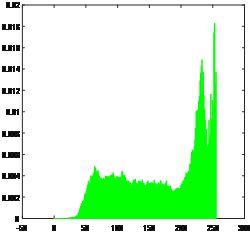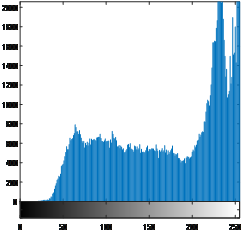2017-04-06 15:42:11 The_star_is_at 阅读数 721
• ###### MATLAB图像处理

MATLAB图像处理课程

4586 人正在学习 去看看 李立宗
```//  Created by liuzhe on 17/3/30.
```

```%-- 17-3-27 上午7:44 --%
%-- 17-3-27 上午7:49 --%
figure(1),imshow(I_huidu);
figure(2),imshow(I_erzhi);
figure(3),imshow(I_erzhi2);
figure(4),imshow(I_suoyin,colormap);
figure(5),imshoew(I_suoyin);
figure(5),imshow(I_suoyin);
figure(5),imshow(colormap);
figure(6),imshow(I_RGB);
R=I_RGB(:,:,1);
figure(100),imshow(R);
G = I_RGB(:,:,2);
B = I_RGB(:,:,3);
figure(G),imshow(G);
figure(101),imshow(G);
figure(102),imshow(B);
subplot(2,2,1),imshow(I_RGB);
subplot(2,2,2),imshow(R);
subplot(2,2,3),imshow(G);
subplot(2,2,4),imshow(B);
GA = imnoise(I,'gaussian');
imwrite(GA,'GApout.tif');
subplot(1,3,1),imshow(I);
subplot(1,3,2),imshow(GA);
PS = imnoise(I,'salt & pepper');
subplot(1,3,3),imshow(PS);
imwrite(PS,'PSpout.tif');
```

2018-04-10 00:00:51 weixin_39569242 阅读数 2572
• ###### MATLAB图像处理

MATLAB图像处理课程

4586 人正在学习 去看看 李立宗

1）掌握点运算和代数运算的算法实现和概念

2）掌握和几何运算的算法实现和概念

2）掌握灰度变换和几何变换的基本方法

3）理解图像灰度直方图的概念

1）任意选择几幅图像，查看其直方图，说明直方图和图像的对应关系。

2）任意选择几幅图像，对其进行灰度线性变换，结合以下情况分析输入图像和输出图像两者有何变化。

Ø  当斜率a>1时；

Ø  当斜率 a<1时；

Ø  当斜率a1b<0时；

Ø  当斜率a1b>0时；

Ø  当斜率a1b=0时；

Ø  当斜率a-1b=255时；

3）选择几幅图像，对其进行灰度拉伸，选择不同的拉伸参数，观察图像与原图有何不同，总结灰度拉伸的原理。

4）选择几幅图像对其进行几何变换，理解不同变换对图像产生的影响。

1代码：

I=rgb2gray(I); %真彩色图像转换为灰度图像，即灰度化处理

for n=0:255

end;

figure; imshow(I);

figure;  bar(0:255,final,'g')

figure; imhist(I)1-1：原图                                                      1-2：灰度图1-3 直方图                                                        1-4 纵坐标扩大直方图

2代码

y=a*I+b/255;    %线性点运算

figure   subplot(2,2,1);    imshow(y);2-1  原图

a,b值分别如下，及结果图:

a=2;b=10;                             a=0.3;b=1;2-2：a>1                                 2-3a<1

a=1;b=-10;             a=1;b=10;              a=1;b=0;2-4a1b<0        2-5a1b>0          2-6a1b=0

a=-1;b=255;图2-7a-1b=255

 如果a>1，输出图像的对比度变大，即图像变得更亮

 如果a<1，输出图像的对比度减小，即图像变得更暗

 如果a=1,b<0,输出图像下移，图像显示的更暗

 如果a=1,b>0,输出图像上移，图像显示的更亮

 如果a=1,b=0,输出图像不变，与原图像一样

 如果a=1,b<0,输出图像下移，图像显示的更暗

 如果a<0,b<0,输出图像亮区变暗，暗区变亮

3代码：

Y=double(I);  %将参数I转为双精度浮点类型

[M,N]=size(Y);

for i=1:M

for j=1:N

if Y(i,j)<=30

Y(i,j)=I(i,j);

else if Y(i,j)<=150

Y(i,j)=(200-30)/(160-30)*(I(i,j)-30)+30;

else   Y(i,j)=(255-200)/(255-150)*(I(i,j)-150)+200;

end

end

end

end

figure(2);     imshow(uint8(Y))3-1：原图                                     图3-2：灰度拉伸

4代码：

se = translate(strel(1), [100 100]);

a = imdilate(I,se);     imshow(a)%平移      figure;

b = imresize(I,1.5)     imshow(b)%放大1.5   figure;

c= imresize(I,0.5)      imshow(c)%缩小0.5    figure;

[height,width,dim]=size(I);

tform1=maketform('affine',[-1 0 0;0 1 0;width 0 1]);

d1=imtransform(I,tform1,'nearest');  imshow(d1);%水平镜像 figure;

tform2=maketform('affine',[1 0 0;0 -1 0;0 height 1]);

d2=imtransform(I,tform2,'nearest');  imshow(d2);%垂直镜像 figure;

e1 = imrotate(I,90);  imshow(e1)%旋转90    figure;

e2 = imrotate(I,180); imshow(e2)%旋转180   figure;

e3 = imrotate(I,270);  imshow(e3)%旋转270  figure;

e4 = imrotate(I,360);  imshow(e4)%旋转360  figure;图4-1:原图                                  图4-2：平移图4-3：放大1.5                                                4-4：缩小0.5图4-5：水平镜像                                4-5：垂直镜像4-7：旋转90度                                       4-8：旋转180图4-9：旋转270度                            4-10：旋转360度

2018-01-06 18:47:37 aninstein 阅读数 14063
• ###### MATLAB图像处理

MATLAB图像处理课程

4586 人正在学习 去看看 李立宗

## 前两节都是熟悉一下怎么在matlab底下对图片做一些操作，并没有什么卵用，这一节稍微有点卵用，灰度变换一般是图像处理的第一步。```% --- Executes on button press in 打开位图.
function openFile_Callback(hObject, ~, handles)
[filename,pathname]=uigetfile('*.bmp','select image');
str=[pathname filename];
guidata(hObject,handles);
axes(handles.axes1);
imshow(handles.I,handles.map);```

```% --- Executes on button press in 转灰度图.
function togray_Callback(hObject, eventdata, handles)
X=rgb2gray(handles.I);%就是这个函数把图片变成灰度图
guidata(hObject,handles);
axes(handles.axes3);
imshow(X,handles.map);```### 1．实现灰度直方图。

```% --- Executes on button press in 显示灰度直方图.
function grayBar_Callback(hObject, eventdata, handles)
X=rgb2gray(handles.I);
axes(handles.axes2);
imhist(X);```

### 2．实现灰度线性变换。

```% --- Executes on button press in 线性变换.
function lineTransfrom_Callback(hObject, eventdata, handles)
X=rgb2gray(handles.I);
axes(handles.axes2);
imshow(uint8(J));```### 3．实现灰度非线性变换。

（1）负相变换

（2）二值化和阈值处理

（3）分段线性变换（4）对数变换，顾名思义就是以对数的函数图像作为变换规则。

```% --- Executes on button press in 对数变换.
function logTransfrom_Callback(hObject, eventdata, handles)
X=rgb2gray(handles.I);
J=double(X);
H=(log(J+1))/10;
axes(handles.axes2);
imshow(H,handles.map);```### 4．实现阈值变换。

```% 阈值输入
function threshold_Callback(hObject, eventdata, handles)
handles.user_entry = str2double(get(hObject,'string'));% 从编辑框中取出阈值，变为数值型
if isnan(handles.user_entry)
uicontrol(hObject)
return
end
guidata(hObject,handles)```

```% --- Executes on button press in 确认阈值并且进行阈值变换.
function confirmThreshold_Callback(hObject, eventdata, handles)
X=rgb2gray(handles.I);
J=X>handles.user_entry;
axes(handles.axes2);
imshow(J,handles.map);```### 5．实现均衡变换。

1.归一化灰度频数直方图，得到频率直方图sk
2.用sk计算频率累计直方图tk，
3.tk做取整扩展：tk = int[(L - 1) * tk + 0.5]，将直方图灰度映射尽量满整个灰度取值空间L
4.确定变换映射关系k->tk

5.根据映射关系变换图像灰度值

```% --- Executes on button press in 均衡化.
function Equalization_Callback(hObject, eventdata, handles)
X=rgb2gray(handles.I);
J=histeq(X);%直方图均衡化函数
axes(handles.axes2);
imshow(uint8(J));```2018-01-08 18:41:26 aninstein 阅读数 3686
• ###### MATLAB图像处理

MATLAB图像处理课程

4586 人正在学习 去看看 李立宗

（二）matlab数字图像处理实验-图像的几何变换

2017-03-31 20:46:28 The_star_is_at 阅读数 1392
• ###### MATLAB图像处理

MATLAB图像处理课程

4586 人正在学习 去看看 李立宗

1 实验目的与要求………………………………………………………………… 2

2 实验环境………………………………………………………………………… 2

3实验一般步骤……………………………………………………………………2

4 实验时数………………………………………………………………………………3

5 实验内容和具体要求…………………………………………………………………3

1、实验目的与要求

（1）加深对上课内容的理解。因为缺少实际操作会使同学难以记住，通过多次上机，就能自然地、熟练地巩固数字图像处理的相关技术和方法。

（2）熟悉所用计算机系统和软件的操作方法，也就是了解和熟悉Matlab环境。掌握图像处理工具箱的基本函数和命令。

（3）学会上机调试程序，善于发现错误，并且能很快地排除错误，使程序能正确运行。计算机技术是实践性很强的技术，要求从事这一领域的人不仅能了解和熟悉有关理论和方法，还要求自己动手实现。因此调试程序本身是程序设计课程的一个重要的内容和基本要求，应给予充分的重视。

2、实验环境

3、实验一般步骤

（1）预先准备好上机所需的程序。手编程序应书写整齐，并经人工检查无误后才能上机，以提高上机效率。对程序中自己有疑问的地方，应做出记号，以便在上机时给予注意。

（2）上机输入和调试程序。应该一人一组，独立上机。上机过程中出现的问题，除了是系统的问题以外，一般应自己独立处理，不要轻易举手问教师。尤其对“出错信息”，应善于自己分析判断。这是学习调试程序的良好机会。在程序调试通过后，打印输出程序清单，在运行时要注意在输入不同图像文件时所得到的不同结果。此时应运行几次，分别检查在不同情况下程序是否正确。

（3）上机结束后，应及时整理出实验报告，实验报告包括以下内容：

① 实验目的

② 实验环境

③ 实验内容

④ 数据处理与讨论分析

4、实验时数

5、实验内容和具体要求

%读入灰度图像pout，并显示

figure(1),  imshow(I_huidu) ,  title('灰度图像');

%读入二值图像circles，并显示，注意查看图像矩阵（uint80表示黑，255表示白）

figure(2),  imshow(I_erzhi) ,  title('二值图像');

%读入二值图像blobs，并显示，注意查看图像矩阵（logical0表示黑，1表示白）

figure(2),  imshow(I_erzhi) ,  title('二值图像');

%读入RGB图像football，并显示

figure(3),  imshow(I_RGB) ,  title('RGB图像');

%读入索引图像trees，并显示

figure(4),  imshow(I_suoyin, colormap) ,  title('索引图像');

2、对一个RGB彩色图像，分别抽取其RGB三个分量层，并显示各层图像。

R=A(:, :, 1);

G=A(:, :, 2);

B=A(:, :, 3);

subplot(2, 2, 1),  imshow(A),  title('原始图像');

subplot(2, 2, 2),  imshow(R),  title('R层图像');

subplot(2, 2, 3),  imshow(G),  title('G层图像');

subplot(2, 2, 4),  imshow(B),  title('B层图像');

3、向灰度图像pout中分别加入高斯噪声和椒盐噪声，显示并保存带有噪声的图像。

subplot(1, 3, 1),  imshow(I),  title('原始图像');

G=imnoise(I, 'gaussian');

subplot(1, 3, 2),  imshow(G),  title('高斯噪声图像');

imwrite(G, 'Gpout.tif');

J=imnoise(I, 'salt & pepper', 0.05);

subplot(1, 3, 3),  imshow(J),  title('椒盐噪声图像');

imwrite(J, 'Jpout.tif');

(1) 为方便用户学习和使用Matlab图像工具箱，Matlab为用户提供了丰富的图像资源，所使用的图像文件都保存在Matlab安装目录下的\toolbox\images\imdemos子目录下。

(2) 将每次实验中需要使用的图像文件，最好在实验开始前事先拷贝到Matlab安装目录下的\work子目录下。（因为Matlab默认处理当前工作目录下的图像文件）

(3) 实验过程中，学会使用help命令。

（1）计算并绘制原始pout图像的灰度直方图；

（2）根据离散累计分布函数，对原始灰度直方图进行均衡化处理，绘制均衡化后的灰度直方图；

（3）生成均衡化处理后的新图像，显示并保存。

（4）比较原始pout图像和新图像的对比度。

clc;

clear;

subplot(2,2,1);  imshow(grayimage);  title('原始图像');       %显示原始图像

[m,n]=size(grayimage);      %获取图像大小

%以下计算原始图像中各灰度级出现的概率

gp=zeros(1,256);  %初始化一维行向量gp

for i=1:256

gp(i)=length(find(grayimage==(i-1)))/(m*n);  %计算每个灰度级出现的概率

end

subplot(2,2,2);  bar(0:255, gp);  %显示原始图像的灰度直方图

title('原始图像的灰度直方图');

xlabel('灰度值');   ylabel('出现概率');

%以下进行直方图均衡化处理，根据离散累计分布函数，求出所有新的灰度级

S1=zeros(1,256);

S2=zeros(1,256);

temp=0;

for i=1:256

temp=temp+gp(i);

S1(i)=temp;

end

S2=round(S1*255);  %Sk归到相近的灰度级上

%以下计算各个新的灰度级出现的概率

newgp=zeros(1,256);

for i=1:256

newgp(i)=sum(gp(find(S2==(i-1))));

end

subplot(2,2,3);  bar(0:255, newgp);  %显示均衡化后的灰度直方图

title('均衡化后的灰度直方图');

xlabel('灰度值');  ylabel('出现概率');

%用新的灰度值填充各像素点，从而获得均衡化处理之后的新图像

newgrayimage=grayimage;

for i=1:256

newgrayimage(find(grayimage==(i-1)))=S2(i);

end

subplot(2,2,4);  imshow(newgrayimage);  %显示均衡化处理后的新图像

title('均衡化后的图像');

imwrite(newgrayimage,'newpout.tif');   %保存均衡化处理后的新图像

1、根据掏空内部点算法，运用Matlab编程实现二值图像的轮廓提取。

%以下适用于黑色背景白色前景的二值图像轮廓提取（以二值图像circles为例）

subplot(1,2,1);  imshow(BW);  title('二值图像');

[M, N]=size(BW);     %M行，N

BW_Buffer=BW;

for i=2: M-1

for j=2: N-1

if (BW(i, j)==255 & BW(i-1, j)==255 & BW(i+1, j)==255 & BW(i, j-1)==255 & BW(i, j+1)==255 & BW(i-1, j-1)==255 & BW(i-1, j+1)==255 & BW(i+1, j-1)==255 & BW(i+1, j+1)==255)    %说明BW(i, j)是前景中的一个内部点

BW_Buffer(i, j)=0;    %掏空该内部点，即将该内部点置成与背景相同灰度

end

end

end

subplot(1,2,2);  imshow(BW_Buffer);  title('提取轮廓');

%以下适用于白色背景黑色前景的二值图像轮廓提取（以二值图像source为例）

subplot(1,2,1);  imshow(BW);  title('二值图像');

[M, N]=size(BW);   %M行，N

BW_Buffer=BW;

for i=2: M-1

for j=2: N-1

if (BW(i, j)==0 & BW(i-1, j)==0 & BW(i+1, j)==0 & BW(i, j-1)==0 & BW(i, j+1)==0 & BW(i-1, j-1)==0 & BW(i-1, j+1)==0 & BW(i+1, j-1)==0 & BW(i+1, j+1)==0)      %说明BW(i, j)是前景中的一个内部点

BW_Buffer(i, j)=255;   %掏空该内部点，即将该内部点置成与背景相同灰度

end

end

end

subplot(1,2,2);  imshow(BW_Buffer);  title('提取轮廓');

2、以灰度图像ricecameraman为例，利用Matlab图像处理工具箱中的edge函数，分别使用Roberts 算子、Sobel算子、Prewitt 算子对其进行边缘检测。

（1）函数格式： BW = edge(I, 'method', thresh)

（2）格式说明：edge函数输入灰度图像矩阵I，输出二值图像矩阵BW；参数'method'用于指定所使用的边缘检测算子，可以是'roberts''sobel''prewitt''log''canny'；参数thresh用于指定梯度门限值（也称梯度阈值），图像中梯度值大于等于门限值thresh的像素用白色（1）表示，说明这些地方对应边缘，梯度值小于门限值thresh的像素用黑色（0）表示（edge function will ignore all edges that are not stronger than thresh）。若不指定参数thresh，则edge函数会自动选择阈值。所以edge函数最终将原始灰度图像中的边缘和背景用二值图像的形式展现出来，以突出边缘的位置，达到边缘检测的目的。

（3）程序如下：

subplot(2,2,1);  imshow(I);  title('原始图像');

[BW1,thresh1]=edge(I,'roberts');  %进行Roberts算子边缘检测并返回门限值

[BW2,thresh2]=edge(I,'sobel');    %进行Sobel算子边缘检测并返回门限值

[BW3,thresh3]=edge(I,'prewitt');  %进行Prewitt算子边缘检测并返回门限值

subplot(2,2,2);  imshow(BW1);  title('Roberts算子边缘检测结果');

subplot(2,2,3);  imshow(BW2);  title('Sobel算子边缘检测结果');

subplot(2,2,4);  imshow(BW3);  title('Prewitt算子边缘检测结果');

subplot(2,3,1);  imshow(I);  title('原始图像');

G=imnoise(I, 'gaussian');  %向原始图像中加入高斯噪声

subplot(2,3,2);  imshow(G);  title('噪声图像');

BW1=edge(G, 'roberts');  %进行Roberts算子边缘检测

BW2=edge(G, 'sobel');    %进行Sobel算子边缘检测

BW3=edge(G, 'prewitt');  %进行Prewitt算子边缘检测

BW4=edge(G, 'log');      %进行Log算子边缘检测

subplot(2,3,3);  imshow(BW1);  title('Roberts算子边缘检测结果');

subplot(2,3,4);  imshow(BW2);  title('Sobel算子边缘检测结果');

subplot(2,3,5);  imshow(BW3);  title('Prewitt算子边缘检测结果');

subplot(2,3,6);  imshow(BW4);  title('Log算子边缘检测结果');

1、以灰度图像cameraman为例，通过Matlab编程实现水平镜像和垂直镜像变换。

subplot(2,2,1);  imshow(I);  title('原始图像');

[M, N]=size(I);    %求出原始图像的高度（行数M）和宽度（列数N

%以下实现水平镜像

I_shuiping=I;

for i=1: M

for j=1: N

I_shuiping(i, j) = I(i, N+1-j);

end

end

subplot(2,2,2);  imshow(I_shuiping);  title('水平镜像图像');

%以下实现垂直镜像

I_chuizhi=I;

for j=1: N

for i=1: M

I_chuizhi(i, j) = I(M+1-i, j);

end

end

subplot(2,2,3);  imshow(I_chuizhi);  title('垂直镜像图像');

subplot(2,2,1);  imshow(I);  title('原始图像');

[M, N, L]=size(I);    %求出原始图像的大小（M行，N列，L=3层）

%以下实现水平镜像

I_shuiping=I;

for k=1:L

for i=1:M

for j=1:N

I_shuiping(i, j, k) = I(i, N+1-j, k);

end

end

end

subplot(2,2,2);  imshow(I_shuiping);  title('水平镜像图像');

%以下实现垂直镜像

I_chuizhi=I;

for k=1:L

for j=1:N

for i=1:M

I_chuizhi(i, j, k) = I(M+1-i, j, k);

end

end

end

subplot(2,2,3);  imshow(I_chuizhi);  title('垂直镜像图像');

2、以灰度图像circuit为例，利用Matlab图像处理工具箱中的imresize函数对图像进行比例缩放变换。

（1）函数格式：

B=imresize(A, m, method)

B=imresize(A, [mrows  ncols], method)

（2）格式说明：A为原始图像矩阵，B为比例缩放变换后的图像矩阵；method指定缩放过程中使用的插值方法，“nearest”为最邻近插值法（默认值），“bilinear”为双线性插值法；m为缩放倍数，m大于1，图像被放大，小于1，图像被缩小；mrowsncols用于分别指定变换后图像的高度和宽度，这能克服缩放倍数m只能对高度和宽度等比例缩放的缺陷。

（3）程序如下：

[M, N]=size(I);       %求出原始图像的高度（行数M）和宽度（列数N

F=imresize(I, 1.5);    %将原始图像等比例放大为原来的1.5

imwrite(F, 'D:\circuit1_5.tif');   %保存放大1.5倍后的图像

[M1_5, N1_5]=size(F);         %求出放大1.5倍后图像的高度和宽度

S=imresize(I, 0.5);             %将原始图像等比例缩小为原来的0.5

imwrite(S, 'D:\circuit0_5.tif');   %保存缩小0.5倍后的图像

[M0_5, N0_5]=size(S);         %求出缩小0.5倍后图像的高度和宽度

J=imresize(I, [190,400]);       %将原始图像缩放为高190400的图像，实现不等比例缩放变换

imwrite(J, 'D:\circuit190_400.tif');   %保存不等比例缩放变换后的图像

3、以灰度图像cameraman为例，利用Matlab图像处理工具箱中的imrotate函数对图像进行旋转变换。

（1）函数格式：

B=imrotate(A, angle)

B=imrotate(A, angle, method)

（2）格式说明：A为原始图像矩阵，B为旋转变换后的图像矩阵；angle指定逆时针旋转角度，当angle为负值时，表示顺时针旋转；method指定旋转过程中使用的插值方法，“nearest”为最邻近插值法（默认值），“bilinear”为双线性插值法。

（3）程序如下：

I_45=imrotate(I, 45);       %将原始图像逆时针旋转45

imwrite(I_45, 'D:\cameraman45.tif');     %保存旋转后的图像

I_45N=imrotate(I, -45);     %将原始图像顺时针旋转45

imwrite(I_45N, 'D:\cameraman45N.tif');     %保存旋转后的图像

I_180=imrotate(I, 180);     %将原始图像逆时针旋转180

imwrite(I_180, 'D:\cameraman180.tif');   %保存旋转后的图像

1avefilt.m

% 自编的均值滤波函数

% x是要进行均值滤波处理的图像矩阵

% n是均值滤波中使用的模板大小（即n×n

% d是均值滤波处理后生成的图像矩阵

function d=avefilt(x, n)

a=ones(n);   % a为大小为n×n的模板，元素全是1，源码a(1:n, 1:n) =1;

[M, N]=size(x);    %图像矩阵为M×N

x1=double(x);

x2=x1;

% A(a:b, c:d)表示取矩阵A的第a行到第b行第c列到第d列的所有元素，构成一个新矩阵

for i=1:M-n+1

for j=1:N-n+1

c=x1(i:i+(n-1), j:j+(n-1)).*a;   %取出x1中从(i, j)开始的nn列元素与模板相乘

s=sum(sum(c));   %c矩阵中所有元素之和

x2(i+(n-1)/2, j+(n-1)/2)=s/(n*n);   %将均值赋给中心元素，未被赋值的元素取原值，即忽略边界像素点的处理

end

end

d=uint8(x2);

--------------------------------------------------------------------------------------------------------------

2junzhilvbo.m

clc;

clear;

G_original=imnoise(original, 'gaussian');

subplot(1,3,1), imshow(original), title('原始图像');

subplot(1,3,2), imshow(G_original), title('加入高斯噪声后的图像');

after=avefilt(G_original, 3);  %调用avefilt函数

subplot(1,3,3), imshow(after), title('均值滤波处理后的图像');

1midfilt.m

% 自编的中值滤波函数

% x是需要进行中值滤波处理的图像矩阵

% n是中值滤波中使用的窗口大小（即n×n

% d是中值滤波处理后生成的图像矩阵

function d=midfilt(x, n)

[M, N]=size(x);   %图像矩阵为M×N

x1=double(x);

x2=x1;

for i=1:M-n+1

for j=1:N-n+1

c=x1(i:i+(n-1), j:j+(n-1));   %取出x1中从(i, j)开始的nn列元素

e=c(1,:);

for k=2:n

e=[e, c(k,:)];   %将矩阵c中的所有元素形成一个行向量

end

x2(i+(n-1)/2, j+(n-1)/2)=median(e);   %将中值赋给中心元素，未被赋值的元素取原值，即忽略边界像素点的处理

end

end

d=uint8(x2);

--------------------------------------------------------------------------------------------------------------

2zhongzhilvbo.m

clc;

clear;

J_original=imnoise(original, 'salt & pepper');

subplot(1,3,1), imshow(original), title('原始图像');

subplot(1,3,2), imshow(J_original), title('加入椒盐噪声后的图像');

after=midfilt(J_original, 3);  %调用midfilt函数

subplot(1,3,3), imshow(after), title('中值滤波处理后的图像');